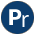#varnumbers.pl -- Utilities for numbered terms

This library provides the inverse functionality of the built-in numbervars/3. Note that this library suffers from the known issues that '\$VAR'(X) is a normal Prolog term and, -unlike the built-in numbervars-, the inverse predicates do not process cyclic terms. The following predicate is true for any acyclic term that contains no '\$VAR'(X), `integer(X)` terms and no constraint variables:

```always_true(X) :-
copy_term(X, X2),
numbervars(X),
varnumbers(X, Copy),
Copy =@= X2.```
See also
- numbervars/4, =@=/2 (variant/2).
Compatibility
- This library was introduced by Quintus and available in many related implementations, although not with exactly the same set of predicates.
numbervars(+Term) is det
Number variables in Term using \$VAR(N). Equivalent to `numbervars(Term, 0, _)`.
See also
- numbervars/3, numbervars/4
varnumbers(+Term, -Copy) is det
Inverse of numbervars/1. Equivalent to ```varnumbers(Term, 0, Copy)```.
varnumbers(+Term, +Start, -Copy) is det
Inverse of numbervars/3. True when Copy is a copy of Term with all variables numbered >= Start consistently replaced by fresh variables. Variables in Term are shared with Copy rather than replaced by fresh variables.
Errors
- `domain_error(acyclic_term, Term)` if Term is cyclic.
Compatibility
- Quintus, SICStus. Not in YAP version of this library
roundup_next_power_two(+Int, -NextPower) is det[private]
NextPower is I**2, such that NextPower >= Int.
max_var_number(+Term, +Start, -Max) is det
True when Max is the max of Start and the highest numbered \$VAR(N) term.
author
- Vitor Santos Costa
Compatibility
- YAP
varnumbers_names(+Term, -Copy, -VariableNames) is det
If Term is a term with numbered and named variables using the reserved term '\$VAR'(X), Copy is a copy of Term where each '\$VAR'(X) is consistently replaced by a fresh variable and Bindings is a list `X = Var`, relating the X terms with the variable it is mapped to.
See also
- numbervars/3, varnumbers/3, read_term/3 using the `variable_names` option.